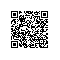# 独家 | kaggle季军新手笔记：利用fast.ai对油棕人工林图像进行快速分类（附代码）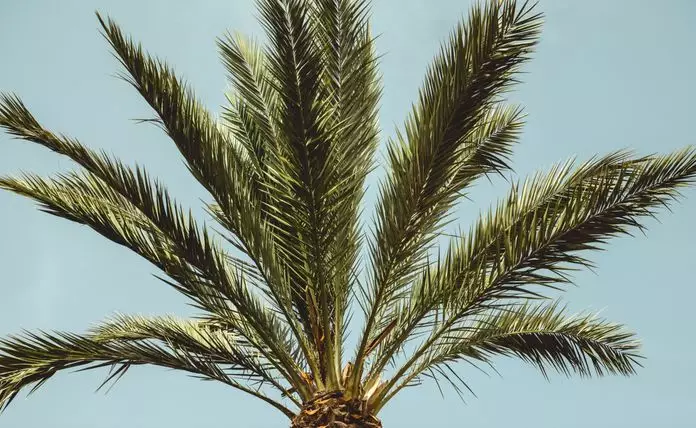WiDS数据马拉松由女性数据科学工作者与她们的伙伴联合发起，她们面临的挑战是需要建立一个模型，来预测一批卫星图像中存在油棕人工林种植园的情况。

### 导入fast.ai和将要用到的其他库

# This ensures that any edits to libraries you make are reloaded here automatically,
# and also that any charts or images displayed are shown in this notebook.
%matplotlib inline导入库
# Import libraries
from fastai import *
from fastai.vision import *
from fastai.callbacks import CSVLogger, SaveModelCallback
import seaborn as sns
import warnings
warnings.filterwarnings('ignore')

### 获取竞赛数据


# Get the data from dropbox link
!wget https://www.dropbox.com/s/6kltw0kqynlijxv/widsdatathon2019.zip

!unzip widsdatathon2019.zip
# The training and testing data have already been seperated, Unzip them as well
!unzip train_images.zip
!unzip leaderboard_test_data.zip

### 查看数据

# Overview of the labels of the training data;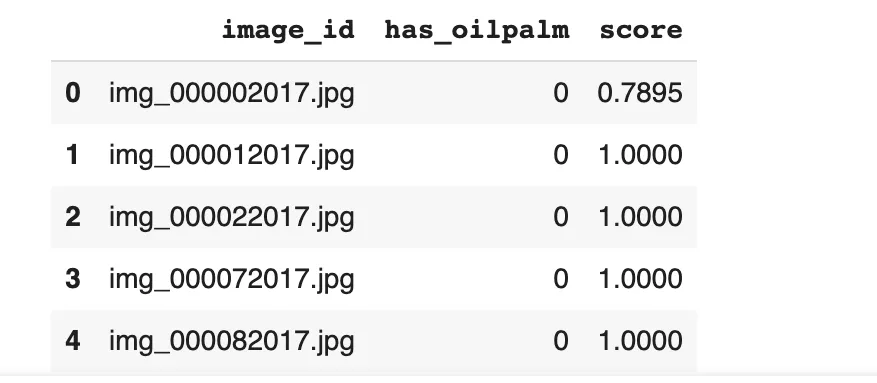sns.countplot(df.has_oilpalm)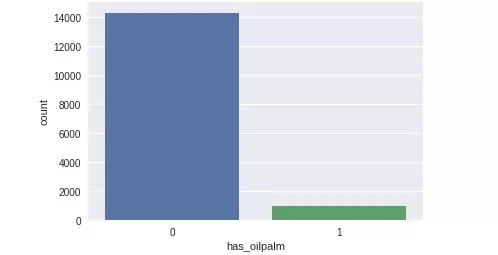### 准备数据

test_imgs = [i for i in test.iterdir()]
hold_imgs = [i for i in lb_test.iterdir()]
combined_test = test_imgs + hold_imgs
len(combined_test)


src = (ImageList.from_df(df, path, folder='train_images')
.random_split_by_pct(0.2, seed=14)
.label_from_df('has_oilpalm')

data = (src.transform(get_transforms(flip_vert=True), size=164)
.databunch()
.normalize(imagenet_stats))


• 利用ImageList的from_df方法来保存训练数据，这样做是因为将有关训练集的信息存储在名字为df的数据帧中，让它能找到训练图像所在的路径和保存图像的文件夹名称，train_images.
• 接下来，使用随机分割来对训练集进行分割，留出20%的数据来监控模型在训练过程中的性能。选择一颗种子，以确保再次检查时能得到同样的结果，我们必须知道什么在起作用，什么不起作用。
• 告诉ImageList在训练集中的数据的标签所在地，利用has_oilpalm方法将组合后的数据添加到测试数据中。
• 最后，对数据进行转换，使用flip_vert = True翻转图像有助于模型识别图像。利用imagenet_stats对图像归一化处理。注意：这是一种转移学习技术，我要说的是需要保持操作尽可能简单。

## 图像预览

data.show_batch(2)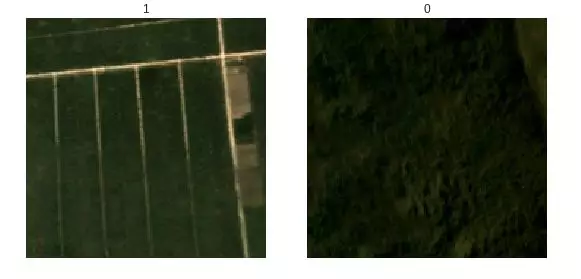## 训练模型

learn = create_cnn(data, models.resnet50, metrics=[accuracy, error_rate],
callback_fns=[ShowGraph, SaveModelCallback])
# View model architecture
learn.model()


learn.lr_find()
learn.recorder.plot()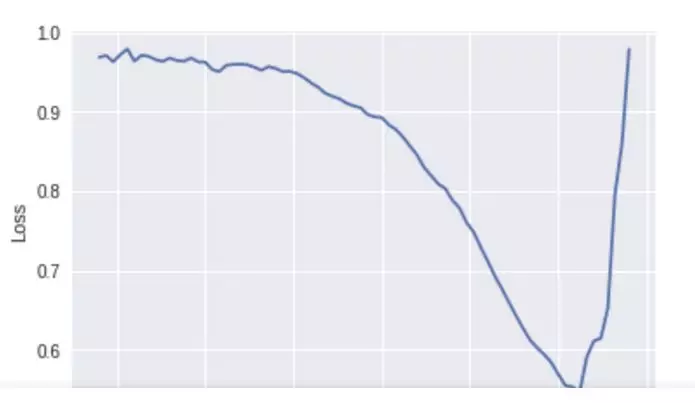learn.fit_one_cycle(5, slice(1e-2))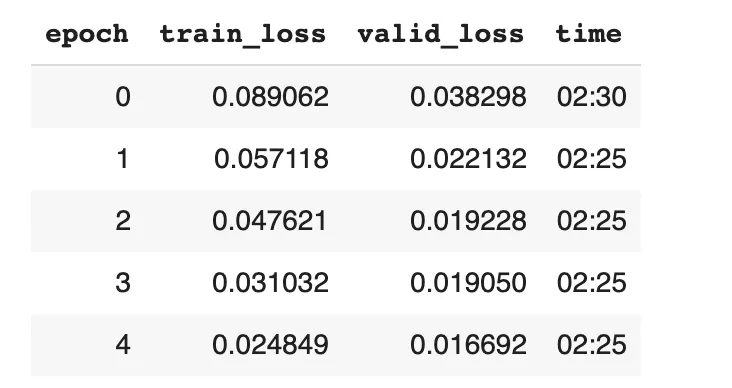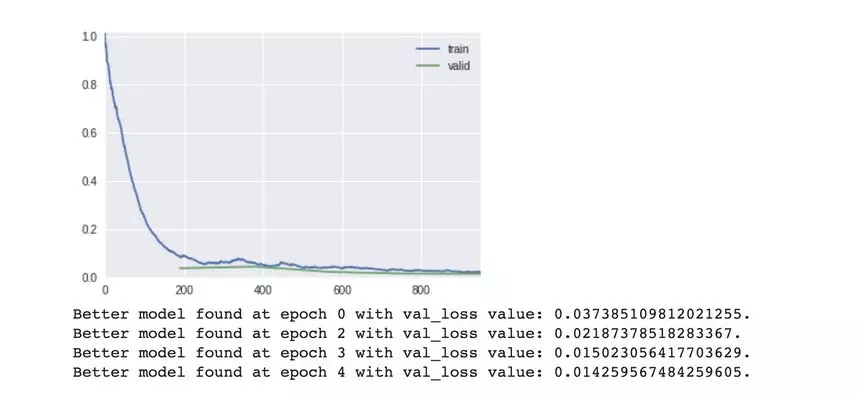fast.ai在运行训练和验证数据集时，内部自动选取和保存最优的那个模型。

## 评估模型

https://www.kaggle.com/learn-forum/5378


from sklearn.metrics import roc_auc_score
def auc_score(y_score,y_true):
probs,val_labels = learn.get_preds(ds_type=DatasetType.Valid)
print('Accuracy',accuracy(probs,val_labels)),
print('Error Rate', error_rate(probs, val_labels))
print('AUC', auc_score(probs,val_labels))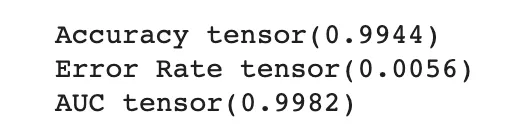learn.save('resnet50-stg1')

interp = ClassificationInterpretation.from_learner(learn)
interp.plot_confusion_matrix(dpi=120)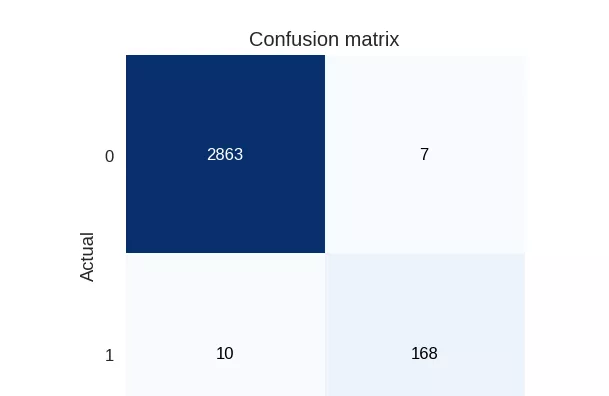learn.lr_find()
learn.recorder.plot()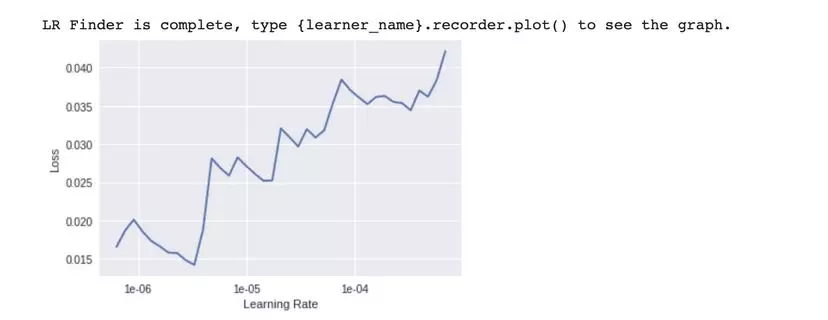learn.fit_one_cycle(7, max_lr=slice(1e-6,1e-4))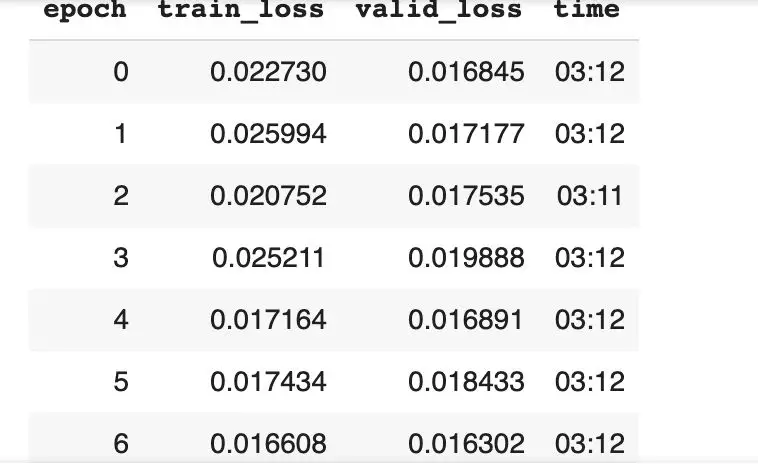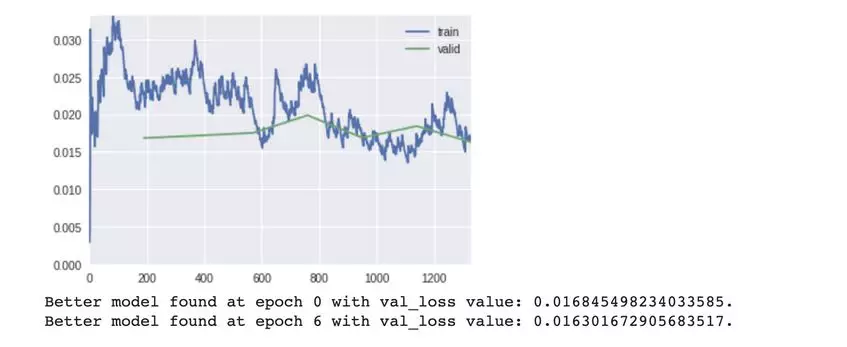learn.save('resnet50-stg2')

probs,val_labels = learn.get_preds(ds_type=DatasetType.Valid)
print('Accuracy',accuracy(probs,val_labels)),
print('Error Rate', error_rate(probs, val_labels))
print('AUC', auc_score(probs,val_labels))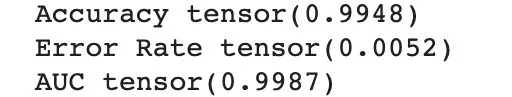interp = ClassificationInterpretation.from_learner(learn)
interp.plot_confusion_matrix(dpi=120)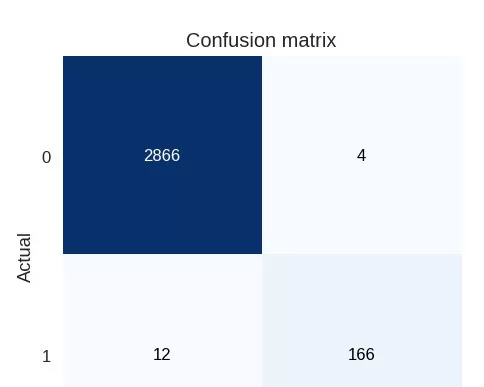## 图像变换

 tfms = get_transforms(flip_vert=True, max_lighting=0.1, max_zoom=1.05, max_warp=0.)
data = (src.transform(tfms, size=200)
.databunch().normalize(imagenet_stats))

learn.data = data

data.train_ds.shape


hting：如果非空 None，则在概率p_lighting下使用由max_light控制的随机亮度和对比度变化。

max_zoom:如果非1，或是一个比1更小的数，则在概率p_affine下使用max_zoom到1之间的随机缩放比

max_warp：如果非空None，则使用概率p_affine应用-max_warp和max_warp之间的随机对称翘曲。

learn.lr_find()
learn.recorder.plot()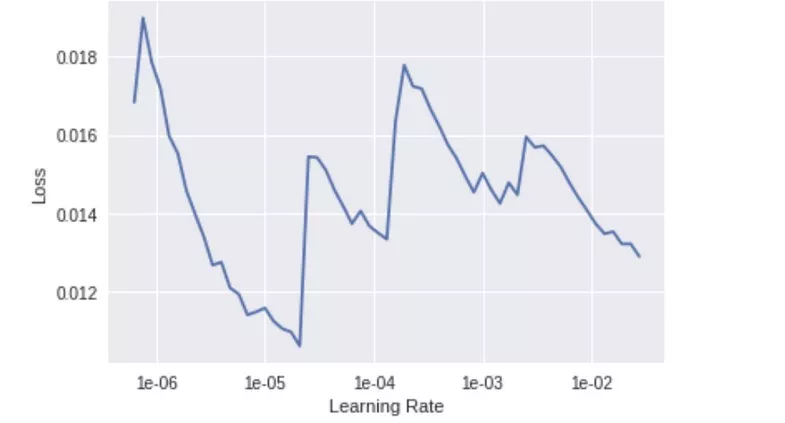learn.fit_one_cycle(5, 1e-6)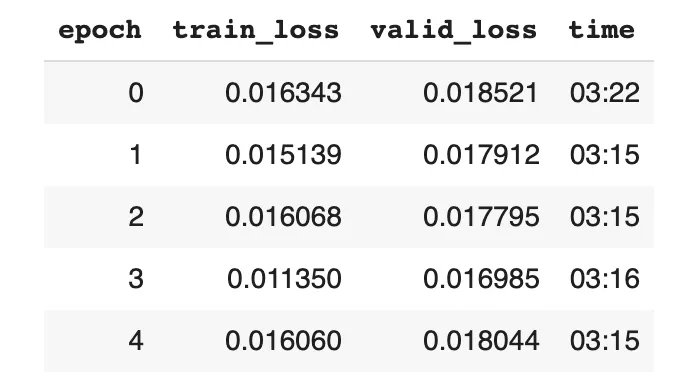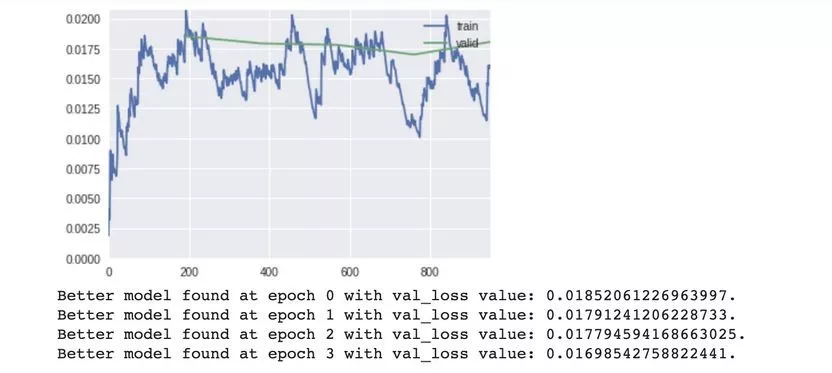learn.save('resnet50-stg3')
probs,val_labels = learn.get_preds(ds_type=DatasetType.Valid)
print('Accuracy',accuracy(probs,val_labels)),
print('Error Rate', error_rate(probs, val_labels))
print('AUC', auc_score(probs,val_labels))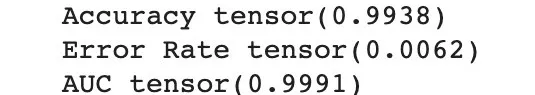## 最终训练阶段


tfms = get_transforms(flip_vert=True, max_lighting=0.1, max_zoom=1.05, max_warp=0.)
data = (src.transform(tfms, size=256)
.databunch().normalize(imagenet_stats))

learn.data = data

data.train_ds.shape



learn.lr_find()
learn.recorder.plot()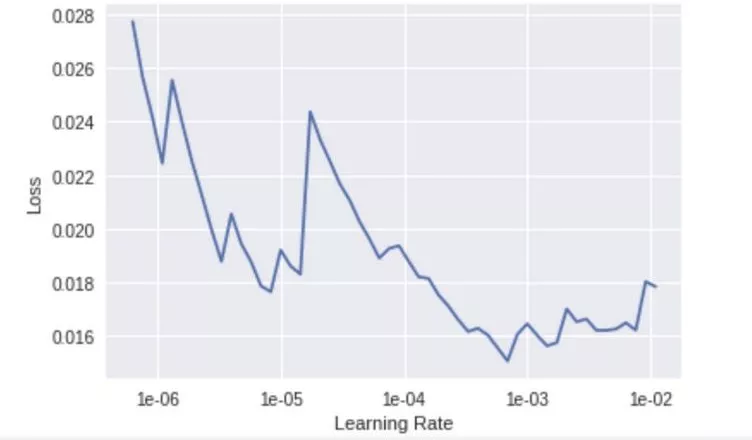learn.fit_one_cycle(5, slice(1e-4))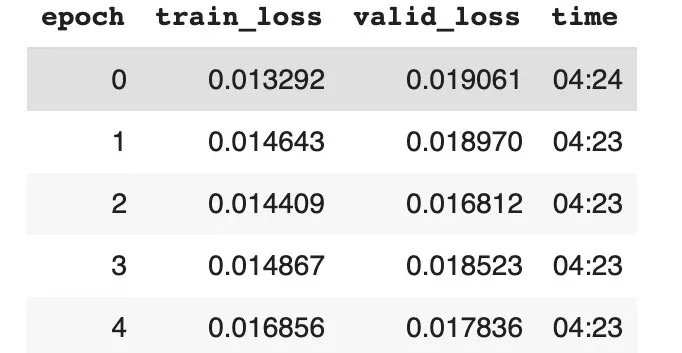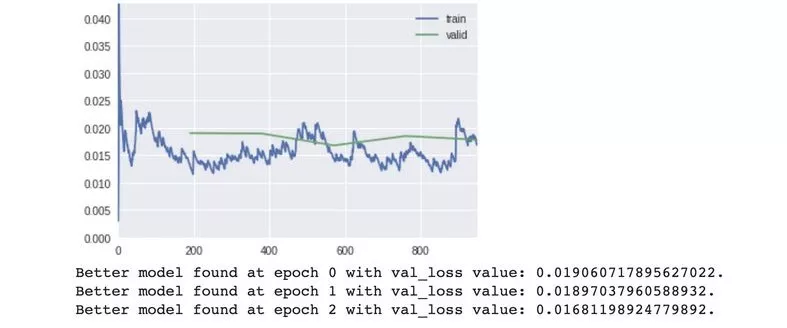learn.save('resnet50-stg4')
probs,val_labels = learn.get_preds(ds_type=DatasetType.Valid)
print('Accuracy',accuracy(probs,val_labels)),
print('Error Rate', error_rate(probs, val_labels))
print('AUC', auc_score(probs,val_labels))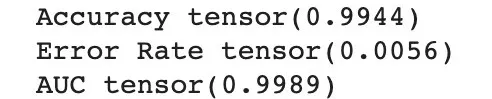## 准备竞赛提交文件

p,t = learn.get_preds(ds_type=DatasetType.Test)

p = to_np(p);
p.shape

ids = np.array([f.name for f in (combined_test)]);
ids.shape

sample_sub = Path('data/SampleSubmission.csv')

sub = pd.DataFrame(np.stack([ids, p[:,1]], axis=1), columns=df_sample.columns)

sub.to_csv(path/'wids-notebook.csv', index=False)


## 向WiDS数据马拉松提交文件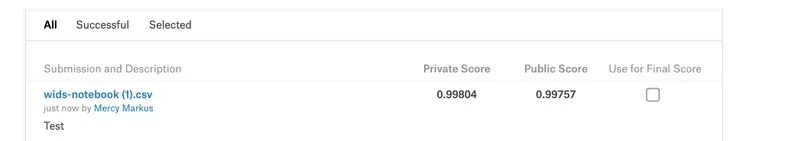How a team of deep learning newbies came 3rd place in a kaggle contest——Classifying images of oil palm plantations using fast.ai

### 译者简介